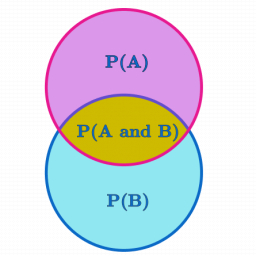# Ordered pairs

Given: Set T = {(1,2), (2,3), (3,4), (4,5), (5,5), (6,7), (6,6), (7,8), (8,9), (9,9), (9, 10), (11,12), (12,13), (13,14), (15,16),
(16,16), (17,18), (18,19), (20,21)} Find the probability of having an ordered pair wherein the second element is greater than the first element.

p =  0.7895

### Step-by-step explanation:Did you find an error or inaccuracy? Feel free to write us. Thank you!

Tips for related online calculators
Need help calculating sum, simplifying, or multiplying fractions? Try our fraction calculator.
Would you like to compute the count of combinations?